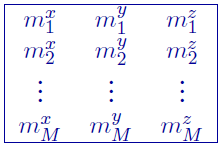# 5.4.4. Vector model file¶

This file contains the three components of property values within the model. The following is the file structure of the model fileEach $$m^p_{i,j,k}$$ is the property in the $$[i,j,k]^{th}$$ model cell where $$p = x, y, z$$ with x-positive easting, y-positive northing, and z-positive down. $$[i, j, k]=[1, 1, 1]$$ is defined as the cell at the top, south-west corner of the model. The total number of lines in this file should equal $$NN \times NE \times NZ$$, where $$NN$$ is the number of cells in the north direction, $$NE$$ is the number of cells in the east direction, and $$NZ$$ is the number of cells in the vertical direction. The model ordering is performed first in the z-direction (top-to-bottom), then in the easting, and finally in the northing.